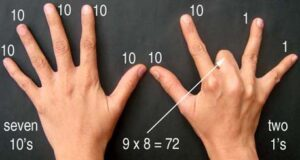# Finger multiplication for a table of 9

~saranya

We are going to learn finger multiplication for a table of 9: Fingers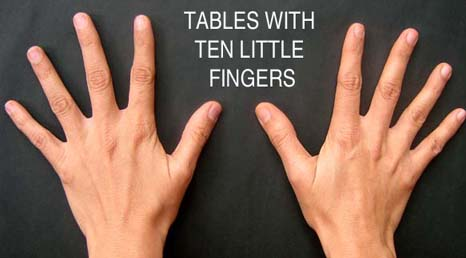Fingers to the left of the closed finger represent 10’s and the remaining fingers 1’s.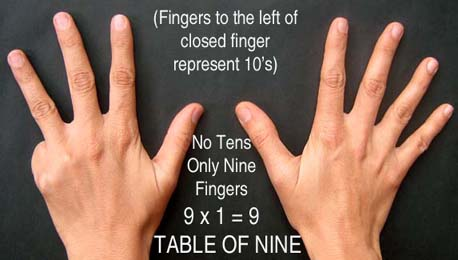In the below picture: fingers to the left of the closed finger are 1 which means 1 ten and 8 ones are there. It represents 9×2=18.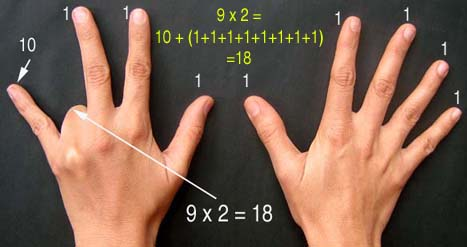In the below picture: fingers to the left of the closed finger are 6 which means 6 ten and 3 ones are there. It represents 9×7=63.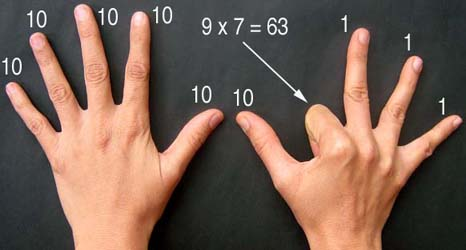Similarly, fingers to the left of the closed finger are 7 which means 7 ten and 2 ones are there. It represents 9×8=72.